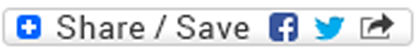#### A Brief Course in Linear Algebra

##### Matrices and Matrix Equations for Undergraduate Students in Applied Mathematics, Science and Engineeringby Whye-Teong Ang• Number of Pages: 204
• ISBN-10: 1627347240
• ISBN-13: 9781627347242
• Publisher: BrownWalker Press
• Year: 2019
• Category: Mathematics, Mathematics

# Synopsis

This book contains a brief course in elementary linear algebra with emphasis on solving systems of linear algebraic and ordinary differential equations. It is written for undergraduate students in the disciplines of applied mathematics, science and engineering. Topics covered include basics of matrices and vectors, systems of linear algebraic equations, matrix inverse, matrix determinant, eigenvalues and eigenvectors, matrix diagonalization, and systems of first order linear ordinary differential equations. The connections between the topics are carefully elucidated. Derivations or proofs are given in detail for all the main results in the book. Basic knowledge of the arithmetic of complex numbers and exposure to elementary functions and calculus are assumed. Problems are set at the end of every main chapter of the book to test the understanding of students and to provide further insights into the topics studied. View or Post a Review at Amazon.com

W. T. Ang graduated with a PhD in Applied Mathematics from the University of Adelaide in Australia. He has considerable experience in tertiary teaching of applied and engineering mathematics. He is currently affiliated with Nanyang Technological University in Singapore.

## Printed Edition

Order from Universal-Publishers

QTY